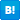コメントを書くコメントを見るＣＤ楽譜Check作詞 FREKAS OF GOGO SPECTATORS 作曲 FREAKS OF GO GO SPECTATORS 唄 SpiralLife
you could find the answer ウォー
you could find the answer, baby you know

you could find the answer ウォー
you could find the answer, baby
you could find the answer ウォー
you could find the answer, baby

これで僕の時間計る ウォー

you could find the answer ウォー
you could find the answer, baby
you could find the answer ウォー
you could find the answer, baby you know

oh, colouredの壁 与えられたキャンディー
どこへ行こうとする 無限と壁のチャーミング
そう見えかくれする ひとりよがりのunder the messages
もうかすかな夢 溶けて消えてくスマイル

ひとコマずつ進んでいく矛盾の全て すべての神 すべての時空 ウォー

you could find the answer ウォー
you could find the answer, baby

you could find the answer ウォー

you could find the answer, baby
you could find the answer ウォー
you could find the answer, baby
you could find the answer ウォー
you could find the answer, baby
you could find the answer ウォー
you could find the answer, baby
you could find the answer ウォー
you could find the answer, baby
you could find the answer ウォー

you could find the answer, baby

うたまっぷ 」では、著作権保護の観点より歌詞の印刷行為を禁止しています。

words by フリークスオブゴーゴースペクテイターズ
music by フリークスオブゴーゴースペクテイターズ
Performed by スパイラルライフ